#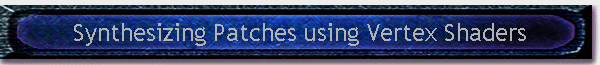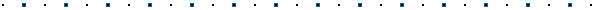Written by Robert Dunlop Microsoft DirectX MVPPage 1 Next: Page 2 >## Introduction

DirectX 8 Graphics introduced programmable vertex shaders, which allows the vertex processing of Direct3D to be replaced by application specific code.  This code, known as a vertex shader, performs the transformation and lighting of vertices, determining their final position, color parameters, and texture coordinates.

In this article, we will examine a novel method for rendering landscapes, which uses a vertex shader to perform real-time modeling of bicubic patches in the 3D pipeline.

## Catmull-Rom Splines

Splines are a mathematical means of representing a curve, by specifying a series of points at intervals along the curve and defining a function that allows additional points within an interval to be calculated.  There are various functions available to approximate a curve, but in this article we will focus on the Catmull-Rom spline.

The points that define the curve are known as "Control Points".  One of the features of the Catmull-Rom spline is that the specified curve will pass through all of the control points - this is not true of all types of splines.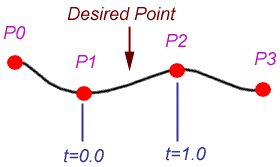Figure 1 To calculate a point on the curve, two points on either side of the desired point are required, as shown on the left.  The point is specified by a value t that signifies the portion of the distance between the two nearest control points.

Given the control points P0, P1, P2, and P3, and the value t, the location of the point can be calculated as (assuming uniform spacing of control points):

 q(t) = 0.5 * (1.0f,t,t2,t3)  * [  0 2 0 0 ] [P1] [ -1 0 1 0 ] * [P2] [  2 -5 4 -1 ] [P3] [ -1 3 -3 1 ] [P4] Equation 1

To put that another way:

 q(t) = 0.5 *( (2 * P1) + (-P1 + P3) * t + (2*P1 - 5*P2 + 4*P3 - P4) * t2 + (-P1 + 3*P2 - 3*P3 + P4) * t3) Equation 2

This formula gives Catmull-Rom spline the following characteristics:The spline passes through all of the control points. (see Fig. 1)The spline is C1 continuous, meaning that there are no discontinuities in the tangent direction and magnitude. (See Fig. 2)The spline is not C2 continuous.  The second derivative is linearly interpolated within each segment, causing the curvature to vary linearly over the length of the segment.Points on a segment may lie outside of the domain of P2 -> P3. (See Fig. 2)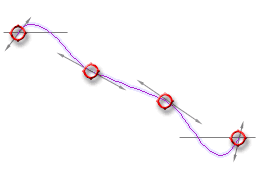Figure 2

While a spline segment is defined using four control points, a spline may have any number of additional control points.  This results in a continuous chain of segments, each defined by the two control points that form the endpoints of the segments, plus an additional control point on either side of the endpoints.  Thus for a given segment with endpoints Pn and Pn+1, the segment would be calculated using [Pn-1, Pn, Pn+1, Pn+2].

Because a segment requires control points to the outside of the segment endpoints, the segments at the extreme ends of the spline cannot be calculated.  Thus, for a spline with control points 1 through N, the minimum segment that can be formulated is P2<->P3, and the maximum segment is PN-2<->PN-1.   Thus, to define S segments, S+3 control points are required.

## Surface Representation with Splines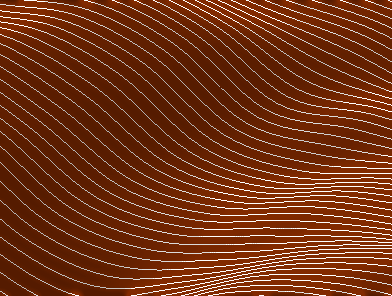Figure 3 Surfaces may be represented as a collection of splines, as illustrated in Figure 3.  Spacing the splines at even distances equal to the control point spacing of the splines results in control points that form a uniform grid. As we discussed earlier, a segment of a spline is defined by four consecutive control points.  Likewise, the control points for parallel segments on four adjacent splines provides the definition for the surface formed between two segments. (See Fig 4)
 The region so defined is known as a bicubic patch, and is defined by 16 control points.  Any point within the patch can be calculated by the same principles applied to the calculation of a point on a spline segment.This can be viewed as a two step process.  Given coordinates of (u,v) within a patch, we first calculate the result for t=u on each of the four splines involved.  This results in four vertices that form a spline passing through the quad, allowing calculation of points on the segment (u,0)<->(u,1).  We then calculate the result of t=v on this on the new spline segment.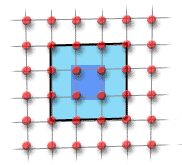Figure 4

To express this algebraically, given function f(t,PA,PB,PC,PD) which calculates a point in a spline segment, then the calculation of a point (u,v) on a surface from control points P1...P16 would be:

f( v, f(u,P1,P2,P3,P4), f(u,P5,P6,P7,P8), f(u,P9,P10,P11,P12), f(u,P13,P14,P15,P16) )

Equation 3

Figure 5, below, illustrates the complete flow of data that occurs when calculating a point in a patch.

 1    u    u2   u3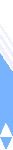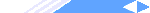• P1  P2  P3  P4 * Basis Matrix * 0.5 -> P'1  P'2   P'3  P'4P5  P6  P7  P8 * Basis Matrix * 0.5 -> P'5  P'6   P'7  P'8 P9  P10 P11 P12 * Basis Matrix * 0.5 -> P'9  P'10' P'11 P'12 1   v  v2  v3 P13 P14 P15 P16 * Basis Matrix * 0.5 -> P'13 P'14  P'15 P'16 • Figure 5 Q1   Q2    Q3   Q4 * Basis Matrix * 0.5 -> Q'1 Q'2 Q'3 Q'4Y at u,v

The processing cost of that operation is quite high to be performed every frame - even given the simplified form presented in Equation 2, the operation would require around 330 floating point operations for each generated 3D vertex.  Because of this, landscape meshes are typically created at load time.  In a large scene, memory footprint can become an issue, so it is may be necessary to store a local area and re-generate portions of a landscape on the fly as the viewer moves into them.  Doing so while maintaining a steady frame rate can require a bit of finesses, so as not to suffer periodic frame rate drops due to processing overhead.

 Page 1 Next: Page 2 >Table of Contents

Page 1

Page 2 Page 3

Introduction

Streamlining the calculations Computing Vertex Position

Catmull-Rom Splines

Using Vertex Shaders to Render Patches Computing Vertex Color

Surface Representation with Splines

Page 4 Page 5
Setting Up the Landscape The Demo Application
Measuring Height at Arbitrary Locations Potential Improvements
Rendering the LandscapeThis site, created by DirectX MVP Robert Dunlop and aided by the work of other volunteers, provides a free on-line resource for DirectX programmers.

Special thanks to WWW.MVPS.ORG, for providing a permanent home for this site.

#####Visitors Since 1/1/2000:Last updated: 07/26/05.